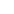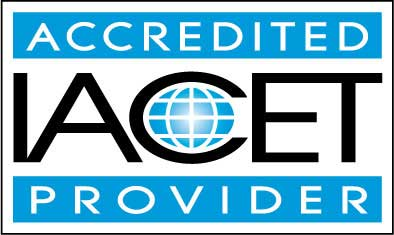# Course Syllabus## Course Description

Fear fractions no more. And learn whether to add first or multiply first in a string of operations. Whether you are struggling with basic math functions in a course today, you are taking a new job that demands understanding of math, or you simply need to review or remember some part of basic math, this course will bring you up to speed. Learn to really understand addition, subtraction, multiplication, and division; know how to calculate and move among decimals, fractions, and percents; figure out ratios and proportions like a pro; and learn to use a calculator for basic math functions.

This course deals with the nuts and bolts of Math. Students will become equipped with a solid foundation of the mathematical tools required to solve more complex mathematical  operations. This course would benefit any student (regardless of age) who requires help understanding basic math skills in order to pass a placement test, a course, a specific job, or a review or brush up on math skills.

No previous education is required though understanding lesson material assumes students at least have a 7th grade reading level. Lessons will focus on the steps involved in adding, subtracting, multiplying, dividing as well as the order of operations. In addition this course will focus on the various forms of numbers such as fractions, decimals, percents, radical signs, absolute value signs exponents, ratios.
• Completely Online
• Self-Paced
• Printable Lessons
• Full HD Video• 6 Months to Complete
• Start Anytime
• PC & Mac Compatible
• Android & iOS Friendly
• Accredited CEUs## Learning Outcomes

By successfully completing this course, students will be able to:
• Summarize numbers and basic terminology.
• Solve subtraction problems.
• Solve multiplication problems.
• Solve division problems.
• Solve problems involving positive and negative numbers.
• Solve fraction problems.
• Solve decimal problems.
• Solve percent problems.
• Demonstrate mastery of lesson content at levels of 70% or higher.

## Assessment Guide

Assessment Points
Introduction Assignment 1 points
Quiz for Lesson 1 - Numbers and Terminology 10 points
Lesson 1 Exercises 10 points
Quiz for Lesson 2 - Addition 10 points
Lesson 2 Exercises 10 points
Quiz for Lesson 3 - Subtraction 10 points
Lesson 3 Exercises 10 points
Quiz for Lesson 4 : Multiplication 10 points
Lesson 4 Exercises 10 points
Quiz for Lesson 5 - Division 10 points
Lesson 5 Exercises 10 points
Quiz for Lesson 6 - Signs used in Operations – Positive and Negative Numbers 10 points
Lesson 6 Exercises 10 points
Quiz for Lesson 7 - Operations and Properties 8 points
Lesson 7 Exercises 9 points
Quiz for Lesson 8 - Fractions 10 points
Lesson 8 Exercises 8 points
Quiz for Lesson 9 - Decimals/The Decimal Number System 9 points
Lesson 9 Exercises 10 points
Quiz for Lesson 10 - Percents 10 points
Lesson 10 Exercises 10 points
Quiz for Lesson 11 - Other Operations 10 points
Quiz for Lesson 12 - Calculators : Using a Calculator 6 points
The Final Exam 140 points